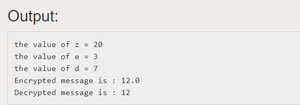Java Program to Implement the RSA Algorithm

• Difficulty Level : Medium
• Last Updated : 27 Apr, 2021

RSA or Rivest–Shamir–Adleman is an algorithm employed by modern computers to encrypt and decrypt messages. It is an asymmetric cryptographic algorithm. Asymmetric means that there are two different keys. This is also called public-key cryptography because one among the keys are often given to anyone. The other is the private key which is kept private. The algorithm is predicated on the very fact that finding the factors of an outsized number is difficult: when the factors are prime numbers, the matter is named prime factorization. It is also a key pair (public and personal key) generator.

Example:

Generating Public Key

1. Select two prime no's. Suppose P = 53 and Q = 59.
Now First part of the Public key  : n = P*Q = 3127.

2. We also need a small exponent say e :
But e Must be

-An integer.

-Not be a factor of n.

-1 < e < Φ(n) [Φ(n) is discussed below],
Let us now consider it to be equal to 3.

The public key has been made of n and e

Generating Private Key

1. We need to calculate Φ(n) :
Such that Φ(n) = (P-1)(Q-1)
so,  Φ(n) = 3016

2. Now calculate Private Key, d :
d = (k*Φ(n) + 1) / e for some integer k
3. For k = 2, value of d is 2011.

The private key has been made of d

Implementation of RSA Algorithm:

1. Consider two prime numbers p and q.
2. Compute n = p*q
3. Compute ϕ(n) = (p – 1) * (q – 1)
4. Choose e such gcd(e , ϕ(n) ) = 1
5. Calculate d such e*d mod ϕ(n) = 1
6. Public Key {e,n} Private Key {d,n}
7. Cipher text C = Pe mod n where P = plaintext
8. For Decryption D = Dd mod n where D will refund the plaintext.

Below is the implementation of the above approach:

Java

 // Java Program to Implement the RSA Algorithmimport java.math.*;import java.util.*; class RSA {    public static void main(String args[])    {        int p, q, n, z, d = 0, e, i;         // The number to be encrypted and decrypted        int msg = 12;        double c;        BigInteger msgback;         // 1st prime number p        p = 3;         // 2nd prime number q        q = 11;        n = p * q;        z = (p - 1) * (q - 1);        System.out.println("the value of z = " + z);         for (e = 2; e < z; e++) {             // e is for public key exponent            if (gcd(e, z) == 1) {                break;            }        }        System.out.println("the value of e = " + e);        for (i = 0; i <= 9; i++) {            int x = 1 + (i * z);             // d is for private key exponent            if (x % e == 0) {                d = x / e;                break;            }        }        System.out.println("the value of d = " + d);        c = (Math.pow(msg, e)) % n;        System.out.println("Encrypted message is : " + c);         // converting int value of n to BigInteger        BigInteger N = BigInteger.valueOf(n);         // converting float value of c to BigInteger        BigInteger C = BigDecimal.valueOf(c).toBigInteger();        msgback = (C.pow(d)).mod(N);        System.out.println("Decrypted message is : "                           + msgback);    }     static int gcd(int e, int z)    {        if (e == 0)            return z;        else            return gcd(z % e, e);    }}My Personal Notes arrow_drop_up